# 9 (1 point) The function f(1) = 11622 is represented as a power series: f(x) =...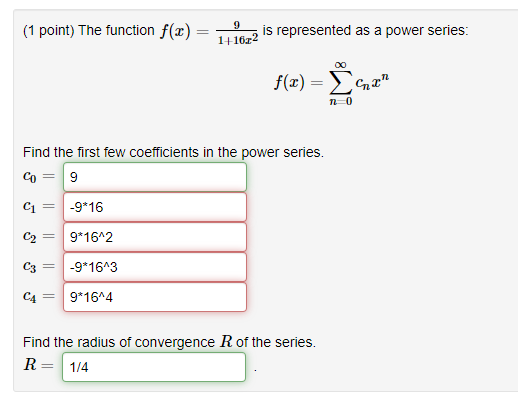9 (1 point) The function f(1) = 11622 is represented as a power series: f(x) = 42" Find the first few coefficients in the power series. Co = 9 C1 = -9*16 C2 = 9*16^2 C3 = -9*1643 9*16^4 Find the radius of convergence R of the series. R= 1/4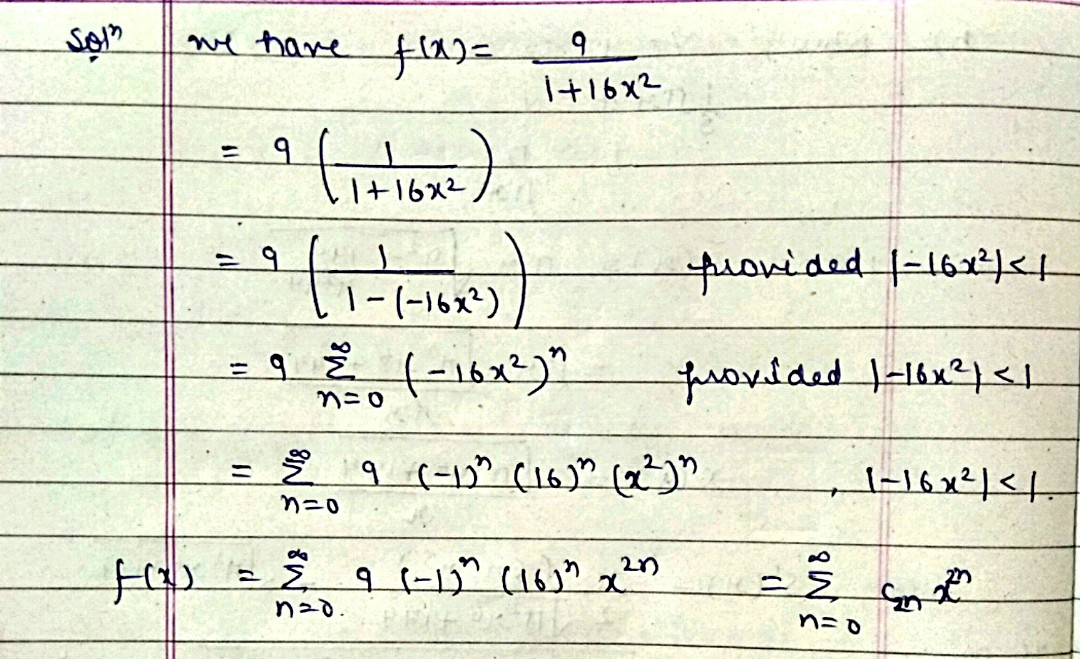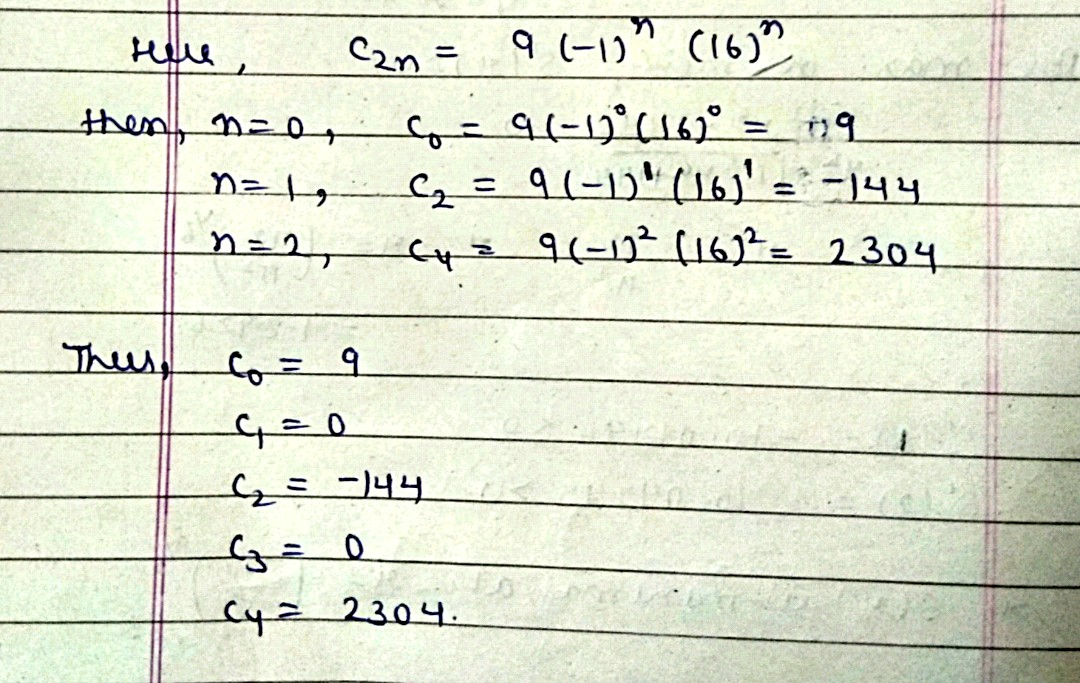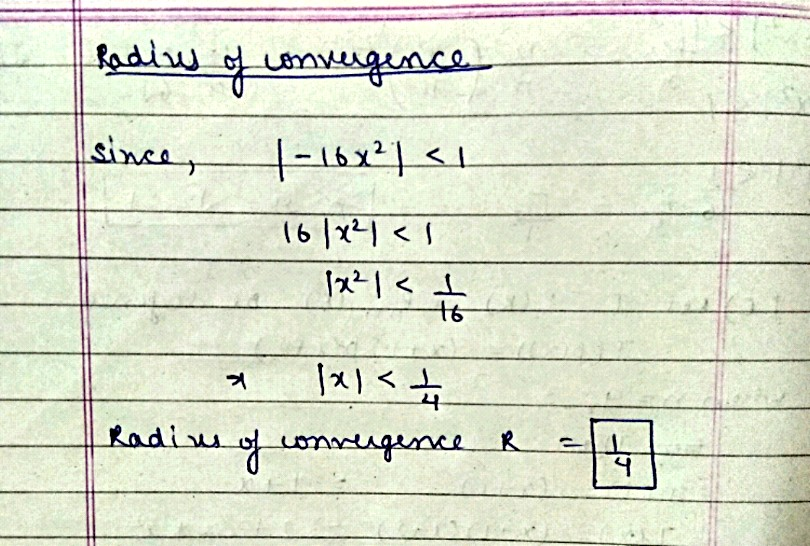#### Earn Coin

Coins can be redeemed for fabulous gifts.

Similar Homework Help Questions
• ### - (1 point) The function f(x) 4 (1-2x)2 is represented as a power series f(x) =...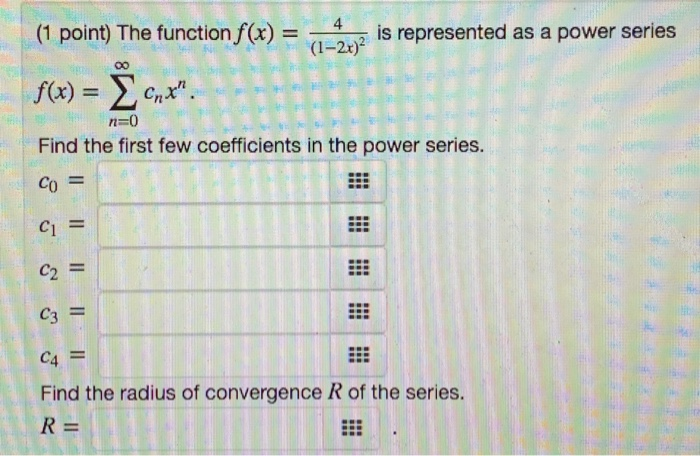- (1 point) The function f(x) 4 (1-2x)2 is represented as a power series f(x) = 0,*". n=0 Find the first few coefficients in the power series. Co = C1 = C2 = C3 = C4 = Find the radius of convergence R of the series. R=

• ### (1 point) The function f(x) = 7 (152) is represented as a power series 00 f(x)...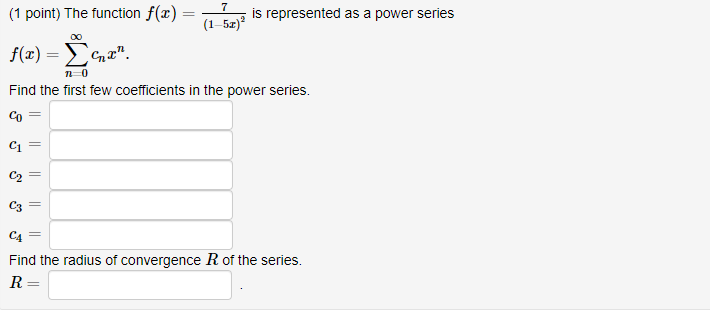(1 point) The function f(x) = 7 (152) is represented as a power series 00 f(x) = 42" 10 Find the first few coefficients in the power series. = C1 C2 = C3 C4 = Find the radius of convergence R of the series. R=

• ### 3 is represented as a power series: (1 point) The function f(x) 1+36x2 Σ f(x) -...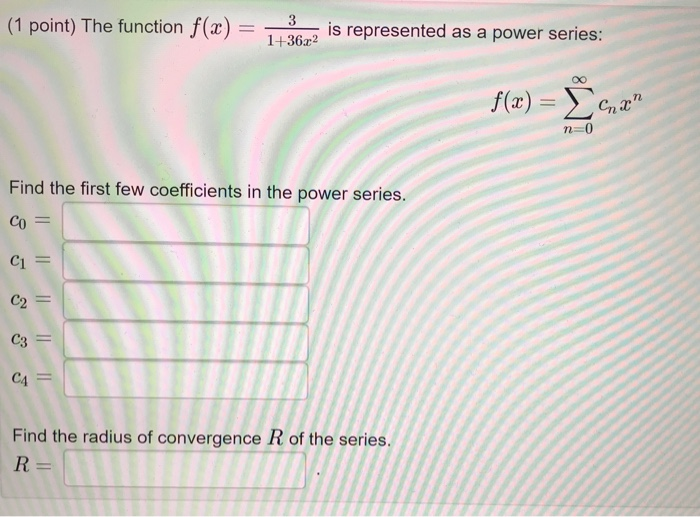3 is represented as a power series: (1 point) The function f(x) 1+36x2 Σ f(x) - n-0 Find the first few coefficients in the power series. CO CI C2 C3 CA Find the radius of convergence R of the series R =

• ### (1 point) The function f(x) = 4x arctan(6x) is represented as a power series f(x) =...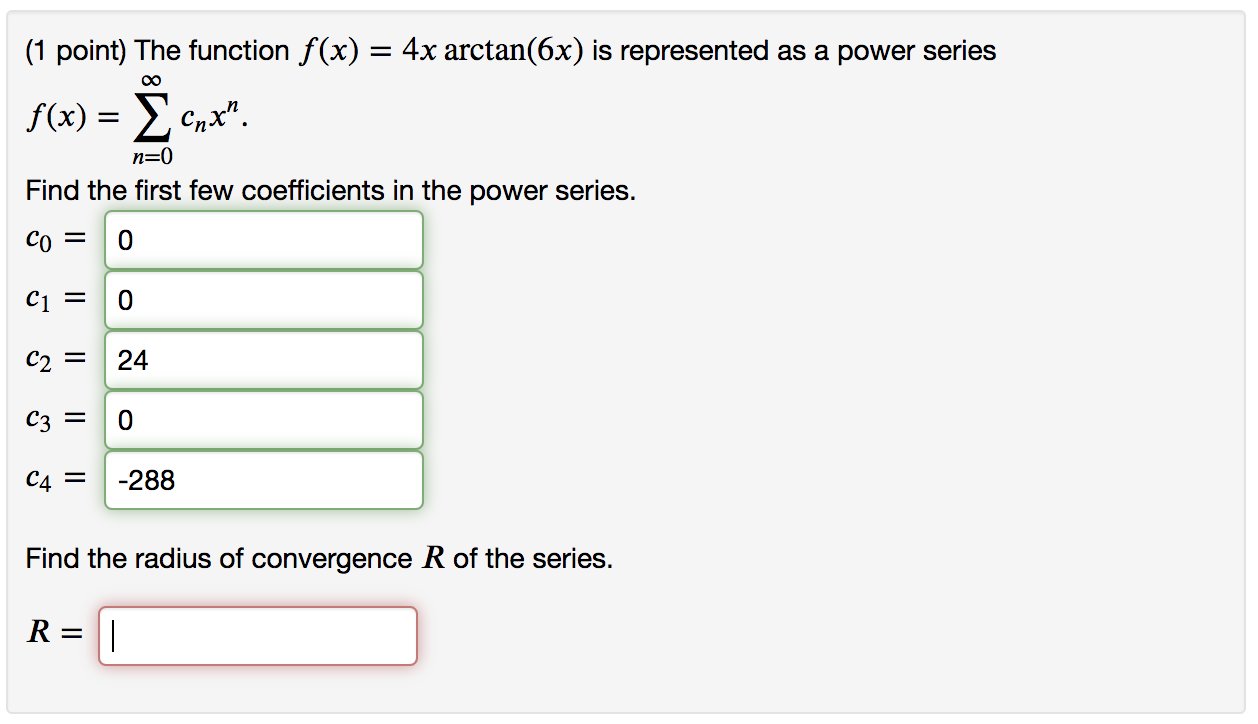(1 point) The function f(x) = 4x arctan(6x) is represented as a power series f(x) = Xcnx". n=0 Find the first few coefficients in the power series. co = 0 Ci = 0 C2 = 24 C3 = 0 C4 = -288 Find the radius of convergence R of the series. R= II

• ### (1 point) The function f(3) = ln(1 – z?) is represented as a power series f(3)...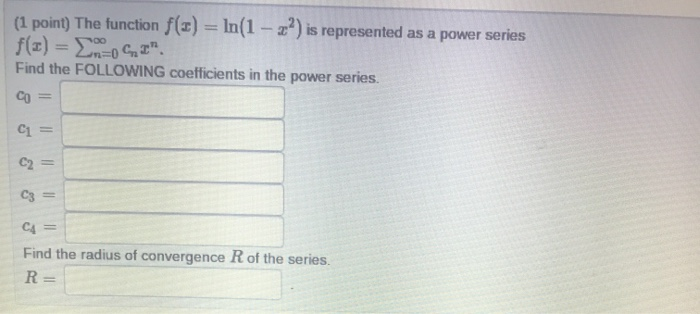(1 point) The function f(3) = ln(1 – z?) is represented as a power series f(3) = EMOCI" Find the FOLLOWING coefficients in the power series. Со Il C1 = C2 = C3 = C4 Find the radius of convergence R of the series. R=

• ### 1. Represent the function 10/1−10x as a power series f(x)=∞∑n=0cn x^n Compute the first few coefficients...

1. Represent the function 10/1−10x as a power series f(x)=∞∑n=0cn x^n Compute the first few coefficients of this power series: c0=    c1=    c2=    c3=    c4= Find the radius of convergence R= 2. The Taylor series for f(x)=e^x at a = 2 is ∞∑n=0 cn(x−2)^n. Find the first few coefficients. c0=    c1=    c2= c3= c4=

• ### 7 Represent the function - as a power series f(x) = { 1 – 40 Chan...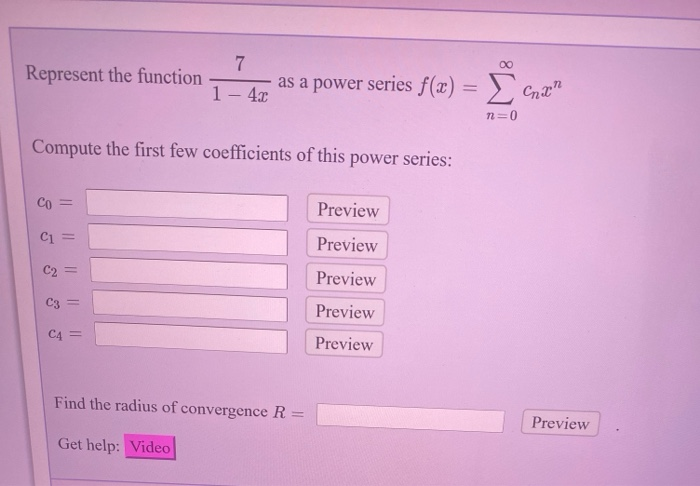7 Represent the function - as a power series f(x) = { 1 – 40 Chan n=0 Compute the first few coefficients of this power series: Co = Preview C1 = Preview C2 Preview C3 = Preview C4 = Preview Find the radius of convergence R = Preview Get help: Video

• ### Express the function as a power series, find the first 5 coefficients of the terms, and...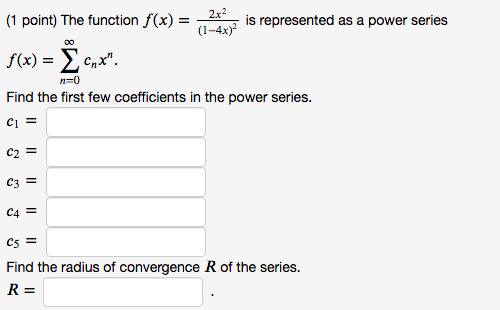Express the function as a power series, find the first 5 coefficients of the terms, and find the radius of convergence. (1 point) The function f(x) = 2x2 is represented as a power series (1-4x) f(x) = Xcnx". Find the first few coefficients in the power series. n=0 Ci = C2 = C3 = C4 = C5 = Find the radius of convergence R of the series. R=

• ### Find the interval of convergence for the given power series. (2 - 4)" 00 n=1 nl...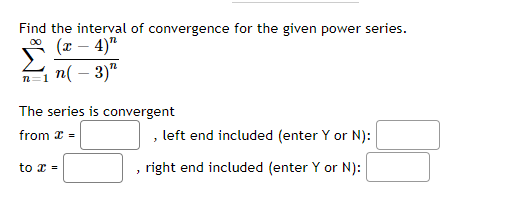Find the interval of convergence for the given power series. (2 - 4)" 00 n=1 nl - 3)" The series is convergent from 2 = , left end included (enter Yor N): right end included (enter Y or N): to C = CI" 10.2 Suppose that (14 + 2) n=0 Find the first few coefficients. Со = C1 C2 C3 C4 Find the radius of convergence R of the power series. R= 2 The function f(x) is represented as a...

• ### 2x (1 point) Represent the function as a power series f(x) = { Cnx" 4 +...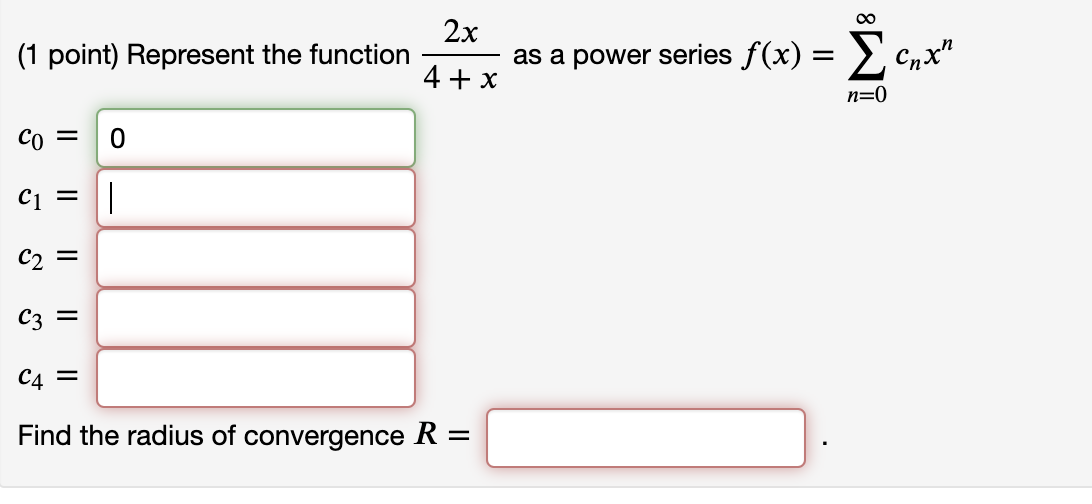2x (1 point) Represent the function as a power series f(x) = { Cnx" 4 + x n=0 Co = 0 C1 = 1 C2 = C3 = C4 = Find the radius of convergence R =